# Algebraic Simplification: Simplifying Expressions in Wolfram|Alpha

April 25, 2011 —Comments Off

Wolfram|Alpha is written in Mathematica, which as its name suggests is a fantastic system for doing mathematics. Strong algorithms for algebraic simplification have always been a central feature of computer algebra systems, so it should come as no surprise to know that Mathematica excels at simplifying algebraic expressions. The main two commands for simplifying an expression in Mathematica are Simplify and FullSimplify. There are also many specific commands for expressing an algebraic expression in some form. For example, if you want to expand a product of linear polynomials, Expand is the appropriate function.

The good news is that everyone has access to the power of Mathematica‘s simplification and algebraic manipulation commands in Wolfram|Alpha. We will now outline some of these features in Wolfram|Alpha, starting with the expression: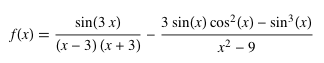and we will use Wolfram|Alpha to break it down to something significantly much simpler.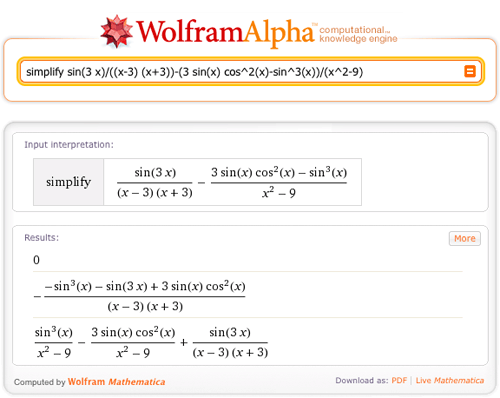The expression simplifies to zero (excluding |x| = 3). Let’s take a closer look at this simplification. First, the denominators. We will expand the polynomial (x – 3)(x + 3) and see that it is equal to the other denominator.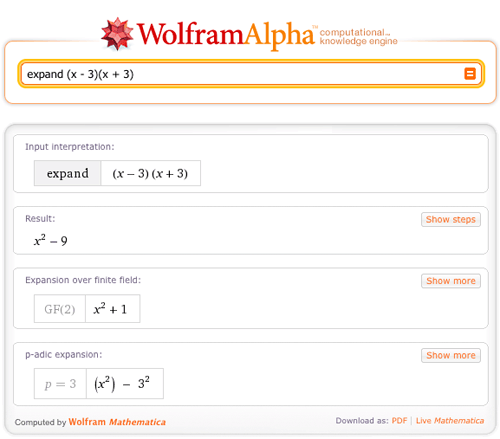So we have a common denominator: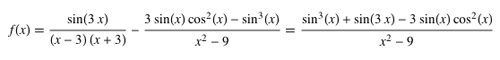Now we can simplify the numerator: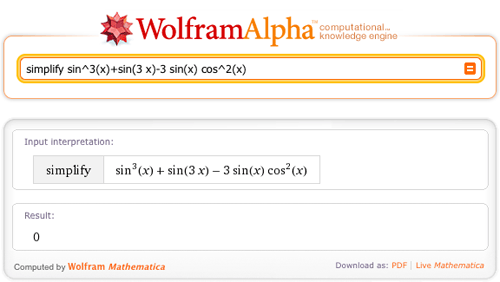We see that it is zero.

Let’s look at some other examples. We can express sin(n x) in terms of a polynomial in sin(x) and cos(x) by asking Wolfram|Alpha: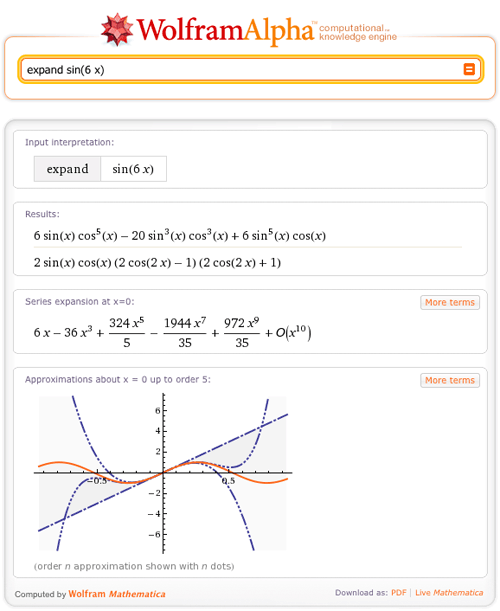We can factor polynomials over their splitting fields: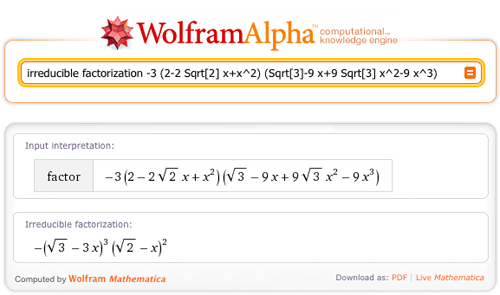Form a single fraction from a number of terms: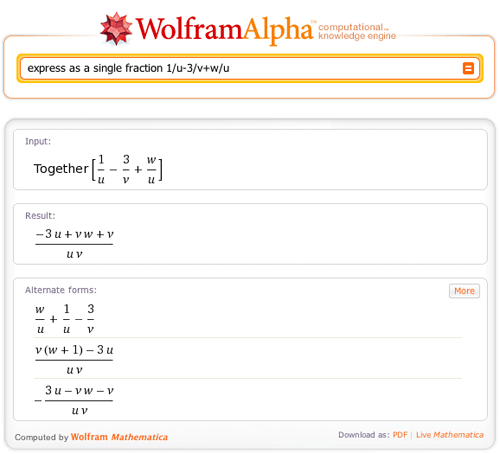Express a single fraction in partial fraction form: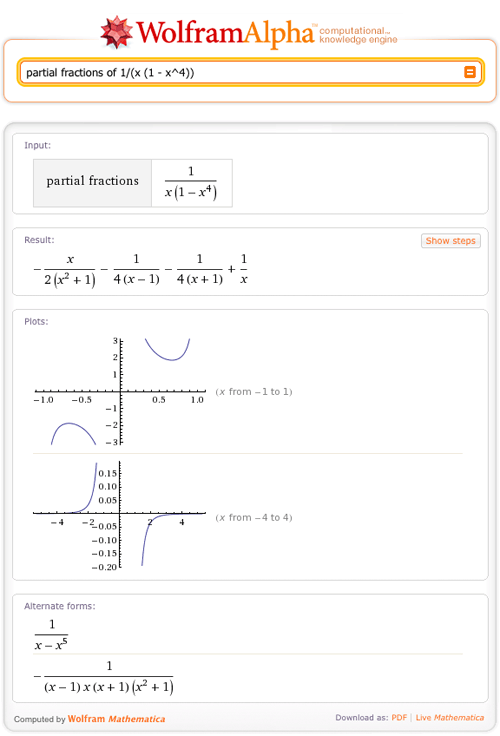And solve equations: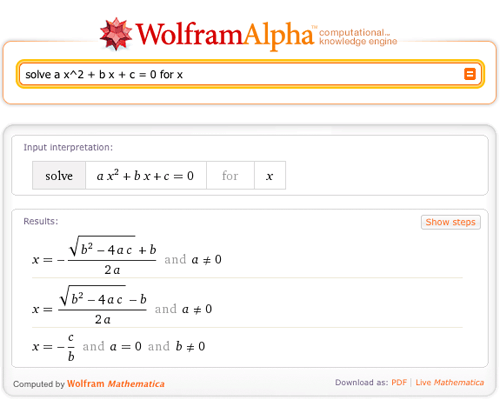We now have implemented a new simplification program for Wolfram|Alpha, which allows Wolfram|Alpha to find even more alternate and simplified forms for algebraic expressions. Here are a couple of examples.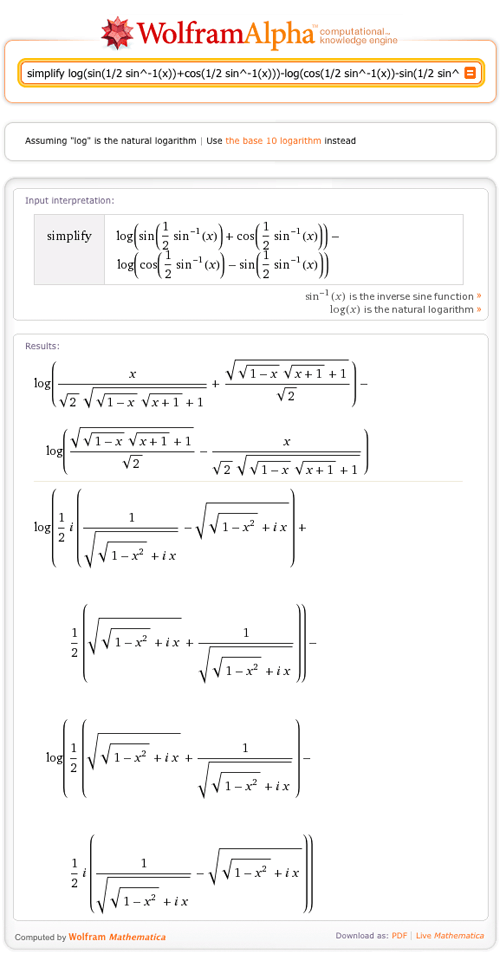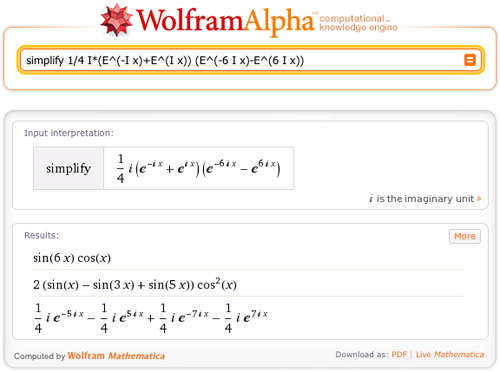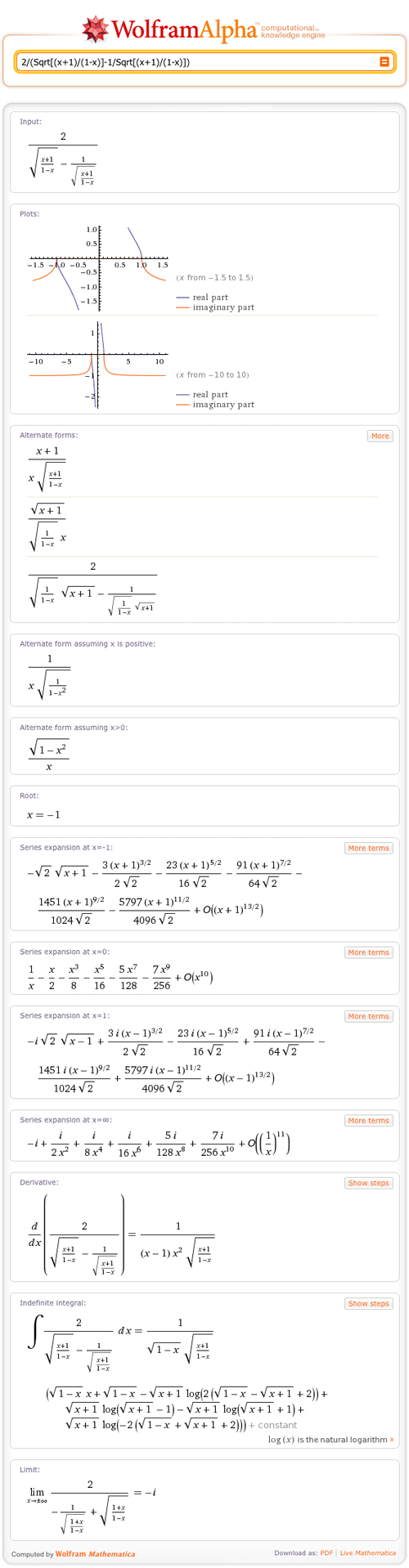In the near future, we will have the functionality to show the steps used to derive an algebraic simplification. Stay tuned to this blog for more details!

Soon, the wolfram alpha will be better than Mathematica itself 😉

Posted by Bartek April 25, 2011 at 12:58 pm

That is an extravagantly helpful new development. Great work, W|A team. Loud applause from my corner!

Posted by Dave Busey April 26, 2011 at 8:05 pm

W|A must be a Scientific Computational Engine.
Wolfram Alpha from the start has been distinguished from search engines in that its answers are to be reliable. This was to arise because its data, including algorithms, was to be curated. It was not said explicitly but the only ssuitable criteria I am aware of are those applied to scientific work. One of the criteria for scientific work is that it is repeatable by other scientists. Thus all W|A output should be repeatable by the user. This requires that users have the option to see all or any of the sources of the data and the logical path leading to W|A’s conclusion.

Posted by Brian Gilbert April 27, 2011 at 1:35 am

W|A team excellent job! Please, when posible try to make this web-blog more smartphone friendly.

Posted by Mauricius GV April 27, 2011 at 2:40 pm

If the number of bracket pairs in an expression exceeds 2 or 3 then it become difficult to sort and pair them visually.
What can be done to facilitate that?
Each pair should have its own color (but colors too are often not distinguishable enough) or a tiny but readable ordinal number or symbol below it. Each pair gets own symbol or ord. number.

An example with 9 pairs:

((a/bc(dxe(f)gh))^i+(jk)*)lm+n)o(pg(r*(s-t*(*uv)))

How quickly can you pair them?

Posted by Vasudev Godbole April 29, 2011 at 8:00 pm

W|A neededs help with solving Elimination standard from lol

Posted by austin May 2, 2011 at 4:21 pm

systems of linear Equations

Posted by austin May 2, 2011 at 4:22 pm

Has my suggestion (of April 29, 2011) about bracket-pairing difficulties in long algebraic expressions with many bracket pairs been discussed? I think it is an easily soluble problem and will make it easier to interpret or work through algebraic expressions.

Posted by Vasudev Godbole August 20, 2011 at 8:20 am

Will there the chance to have a step by step the simplification of expression like 6x*x^(1/2)+3x^2*(1/2)x^(-1/2)?

Posted by Chris October 2, 2014 at 1:20 am